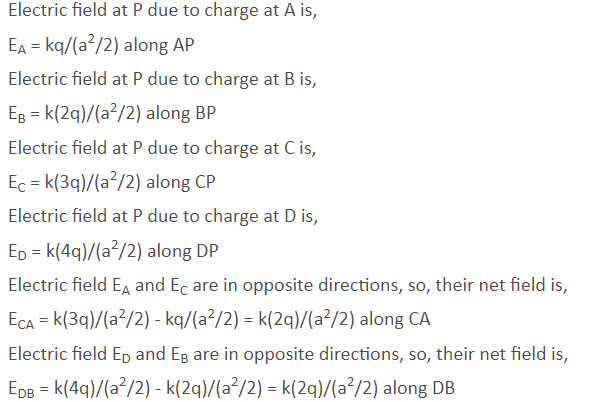# Four charges q,2q,3q and 4q are placed at corners A,B,C and D of a square.The field at the centre of the square has the direction along:OPTIONS: AB CB AC DB AD

4 years ago

Electric field at P due to charge at A is,

EA = kq/(a2/2) along AP

Electric field at P due to charge at B is,

EB = k(2q)/(a2/2) along BP

Electric field at P due to charge at C is,

EC = k(3q)/(a2/2) along CP

Electric field at P due to charge at D is,

ED = k(4q)/(a2/2) along DP

Electric field EA and EC are in opposite directions, so, their net field is,

ECA = k(3q)/(a2/2) - kq/(a2/2) = k(2q)/(a2/2) along CA

Electric field ED and EB are in opposite directions, so, their net field is,

EDB = k(4q)/(a2/2) - k(2q)/(a2/2) = k(2q)/(a2/2) along DB

Since, ECA and EDB are equal in magnitude and they are perpendicular to each other, so, their resultant E will bisect angle APB.

Or we can say it is along CB

2 years ago
Hello studentHope it helps
Thankyou